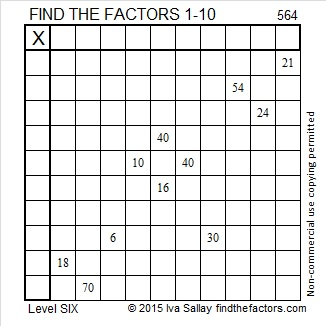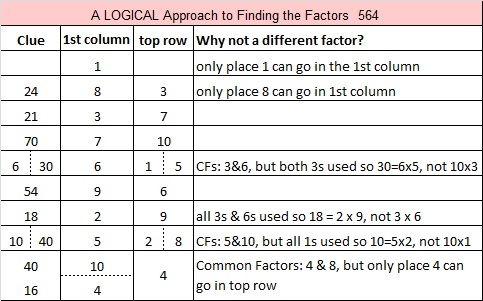# 564 and Level 6

Consecutive primes 281 and 283 add up to 564.

564 is made from three consecutive numbers so it can be evenly divided by 3. If the middle number is divisible by 3, then a number made from three consecutive numbers will also be divisible by 9. Is 564 divisible by 9? Why or why not?Print the puzzles or type the solution on this excel file: 10 Factors 2015-07-20

—————————————————————————————————

• 564 is a composite number.
• Prime factorization: 564 = 2 x 2 x 3 x 47, which can be written 564 = (2^2) x 3 x 47
• The exponents in the prime factorization are 2, 1, and 1. Adding one to each and multiplying we get (2 + 1)(1 + 1)(1 + 1) = 3 x 2 x 2 = 12. Therefore 564 has exactly 12 factors.
• Factors of 564: 1, 2, 3, 4, 6, 12, 47, 94, 141, 188, 282, 564
• Factor pairs: 564 = 1 x 564, 2 x 282, 3 x 188, 4 x 141, 6 x 94, or 12 x 47
• Taking the factor pair with the largest square number factor, we get √564 = (√4)(√141) = 2√141 ≈ 23.74868—————————————————————————————————1.myNeonSun